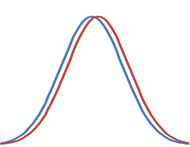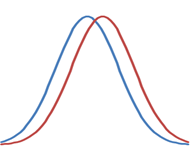## Wednesday, 10 June 2015

### How many times should you run your simulation?

You have a new algorithm, say an evolutionary algorithm.  You want to show it is better than another algorithm.  But its behaviour is stochastic, varying from run to run.  How many times do you need to run it to show what you want to show?  How big a sample of possible results do you need? 10?  100?  1000?  More?

This is a known problem, solved by statisticians, used by scientists who want to know how many experiments they need to run to investigate their hypotheses.

First I recall some statistical terminology (null hypothesis, statistical significance, statistical power, effect size), then describe the way to calculate how many runs you need, using this terminology.  If you know this terminology, you can skip ahead to the final table.

#### null hypothesis

The null hypothesis, H0, is usually a statement of the status quo, of the null effect: the treatment has no effect; the algorithm has the same performance; etc.  The alternative hypothesis, H1, is that the effect is not null.  You are (usually) seeking to refute the null hypothesis in favour of the alternative hypothesis.

#### statistical significance and statistical power

Given a null hypothesis H0 and a statistical test, there are four possibilities.  H0 may or may not be true, and the test may or may not refute it.

H0 true H0 false
refute H0 type I error, false positive correct
fail to refute H0 correct type II error, false negative

The type I error, or false positive, erroneously concludes that the null hypothesis is false; that is, that there is an effect when there is not. The probability of this error (of refuting H0 given that H0 is true) is called α, or the statistical significance level.

α = prob(refute H0|H0) = prob(false positive)

The type II error, or false negative, erroneously fails to refute the null hypothesis; that is, it is a failure to detect an effect that exists.  The probability of this error (of not refuting H0, given that H0 is not true) is called β.

β = prob(not refute H0|not H0) = prob(false negative)

1−β is called the statistical power of the test, the probability of correctly refuting H0 when H0 is not true.

power = 1−β = prob(refute H0|not H0)

Clearly, we would like to minimise both α and β, minimising both false positives and false negatives.  However, these aims are in conflict.  For example, we could trivially minimise α by never refuting H0, but this would maximise β, and vice versa.  The smaller either of them needs to be, the more runs are needed.

Different problems might put different emphasis on α and β.  Consider the case of illness diagnosis, versus your new algorithm.

### Diagnosic test for an illness

• false positive: the test detect illness when there is none, which might result in worry and unnecessary treatment
• false negative: the test fails to detect the illness, which might result in death
So minimising β may take preference in such a case.

### New evolutionary algorithm

• false positive: you claim your algorithm is better when it isn’t; this will be embarrassing for you later when more runs fail to support your claim
• false negative: you fail to detect that your algorithm is better; so you don’t publish
So minimising α may take preference in such a case.

#### p-value

A statistical test results in a p-value.  p is the probability of observing an effect, given that the null hypothesis holds.

p = prob(obs|H0)

A low p-value means a low probability of observing the effect if H0 holds; the conclusion is then that H0 (probably) doesn’t hold.  We refute H0 with confidence level 1−p.

To refute H0 we want the observed p-value to be less than the initially chosen statistical significance α.  A typical confidence level is 95%, or α = 0.05.

#### effect size

The more runs we make, the smaller α and β can be.  That means we can refute H0 at higher and higher confidence level.  In fact, with enough runs we can refute almost any null hypothesis at a given confidence level, since any change to an algorithm probably makes some difference to the outcome.

Alternatively, we can use the higher number of runs to detect smaller and smaller deviations from H0.

For example, if H0 is that the means of the two samples A and B are the same:

H0 : μA = μB

and the alternative hypothesis H1 is that the means are different:

H1 : μA ≠ μB

then with more runs we can detect smaller and smaller differences in the means at a given significance level.  We can measure a smaller and smaller effect size.

Cohen’s d is one measure of effect size for normally distributed samples: the difference in means normalised by the standard deviation:

d = (μA − μB) / σ

So an effect size of 1 occurs when the means are separated by one standard deviation.  Cohen gives names to different effect sizes: he calls 0.2 “small”, 0.5 “medium”, and 0.8 “large” effect sizes.  The smaller the effect size you want to detect, the more runs you need.d = 0.2, “small”d = 0.5, “medium”d = 0.8, “large”

“Small” effect sizes might be fine in certain cases, where a small difference can nevertheless be valuable (either in a product, or in a theory).  However, for an algorithm to be worth publishing, you should probably aim for at least a “medium” effect size, if not a “large” one.

#### sample size

The required sample size, or number of runs, n, depends on your desired values of three parameters: (i) statistical significance α, (ii) statistical power 1−β, and (iii) effect size d.

For two samples of the same size, normally distributed, with H0 : μA = μB, then

n = 2( (z(1−α/2)+z(1−β)) / d)2

where z is the inverse normal cumulative probability distribution function.  In the case of a single sample A compared against a fixed mean value, H0 : μA = μ0, this number can be halved.

A Python script that calculates this value of n (rounded up to an integer) is
from math import ceil
from scipy.stats import norm
n = ceil( 2 * ( (norm.ppf ppf(1-alpha/2) + norm.ppf ppf(power) ) / d ) **2 )

If you want a significance at the 95% level (α = 0.05), and a power of 90%, and want to detect a large effect, then you need 33 runs; if you want to detect a medium effect you need 85 runs, and to detect a small effect you need over 500 runs.  (These numbers are based on the assumption that your samples are normally distributed.  Similar results follow for other distributions.)

At first sight, it might seem strange that the number of runs is somehow independent of the “noisiness” of the algorithm: why don’t we need more runs for an inherently noisy algorithm (large standard deviation) than from a very stable one (small standard deviation)?  The reason is because the effect size is also dependent on the standard deviation: for a given difference in the means, the effect size decreases as the standard deviation increases.

So there you have it.  You don’t have to guess the number of runs, or just do “lots” to be sure: you can calculate n.  And it’s probably less than you would have guessed.